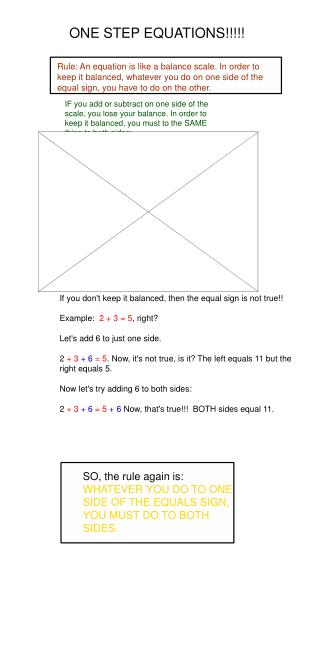# ONE STEP EQUATIONS!!!!! - PowerPoint PPT PresentationDownload PresentationONE STEP EQUATIONS!!!!!

ONE STEP EQUATIONS!!!!!
Download Presentation## ONE STEP EQUATIONS!!!!!

- - - - - - - - - - - - - - - - - - - - - - - - - - - E N D - - - - - - - - - - - - - - - - - - - - - - - - - - -
##### Presentation Transcript

1. ONE STEP EQUATIONS!!!!! Rule: An equation is like a balance scale. In order to keep it balanced, whatever you do on one side of the equal sign, you have to do on the other. IF you add or subtract on one side of the scale, you lose your balance. In order to keep it balanced, you must to the SAME thing to both sides: SO, the rule again is: WHATEVER YOU DO TO ONE SIDE OF THE EQUALS SIGN, YOU MUST DO TO BOTH SIDES. If you don't keep it balanced, then the equal sign is not true!! Example: 2 + 3 = 5, right? Let's add 6 to just one side. 2 + 3+ 6= 5. Now, it's not true, is it? The left equals 11 but the right equals 5. Now let's try adding 6 to both sides: 2 + 3 + 6= 5+ 6 Now, that's true!!! BOTH sides equal 11.

2. One-Step Equations!!! Do you know what the opposite means? Draw a line from each operation to its opposite. multiplication subtraction addition division addition subtraction multiplication division INVERSE is another name for opposite.

3. Next, the rule: to solve a one-step equation, isolate the variable by using the inverse operation. BUT, what does that mean??? Now, for some vocabulary: 1. equation: math sentence with an equals sign. 2. isolate the variable: get the variable alone on one side of the equal sign. 3. solve the equation: find out what the value of the variable is. 4. inverse operation: the opposite operation. To put it simply, if "they" added, we subtract. If they subtracted, we add. If they multiplied, we divide. If they divided, we multiply. THAT IS, we UNDO whatever was done to our variable. For example: x + 3 = 8 THEY added 3, so we subtract 3 (from both sides). x + 3 = 8 -3 -3 x +3 - 3 = 8 -3 x = 5 REMEMBER: if you only do something on one side of the equals sign, you won't be balanced and you'll get the wrong answer!!!

4. PRACTICE: First, let's practice identifying the inverse operation. The first two are done for you. 1) 3x = 8 They multiplied by 3, so we divide by 3 (on both sides). 2) x - 9 = 17 They subtracted 9, so we add 9 (on both sides). Now you try! 3) 6 + x = 189 4) x/4 = 38 5) x + 12,000 = 83,456 6) x - 1/2 = 3 7) 3.75 x = 9.56 8) x/0.8 = 6.1 On the next page, we will begin to USE INVERSE OPERATIONS TO ISOLATE THE VARIABLE.